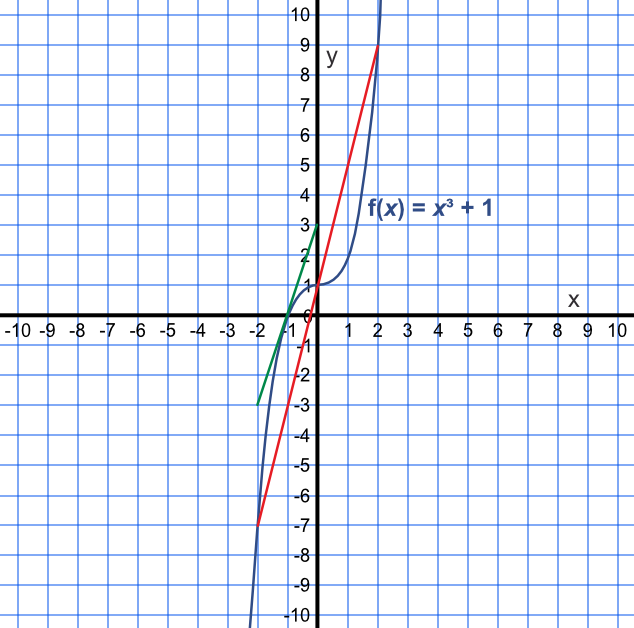Variable Rate of Change

## Variable Rate of Change

A linear function - such as a straight line on a graph - has a constant rate of change. As x changes, so does y, and this is constant. The rate of change can be derived from the gradient:

frac(text(change in )y)(text(change in )x).

For other functions, the rate of change varies over the function: in other words, the gradient on a graph becomes steeper or flatter. At any one point on the graph, the rate of change can be derived (by drawing a tangent to the graph at that point). At a different point, a different rate of change can be found by obtaining the gradient at that point.

## Example 1

A car covers a distance of 20m in 5s. If the car has a constant rate of acceleration, what is the increase in the velocity of the car beween 5s and 10s?

The kinematic formulae are:

v = u + at, s=ut+frac(1)(2)at^2 and v^2=u^2+2as

where u is initial velocity, v is final velocity, s is distance, a is acceleration and t is time.

Known values: s=20, t=5, u=0

 Work out the acceleration s = ut + frac(1)(2)at^2 (20) = (0)(5) + frac(1)(2)a(5)^2 a = frac(8)(5) Velocity at 5s v = u + at v = (0) + frac(8)(5)(5) v = 8 Velocity at 10s v = u + at v = (0) + frac(8)(5)(10) v = 16

Difference in velocity = 16 - 8 = 8ms-1

## Example 2

The gradient of x^3+1 is the same at (-2, -7) as it is at (2, 9). The gradient at (0, 1) is zero.

Jon draws line from (-2, -7) to (2, 9) which passes through (0, 1). Jon states that the rate of change between (-2, -7) and (2, 9) is constant as his line also passes through (0, 1).

Why is Jon wrong?

Draw a tangent at (-1, 0) (or some other point) to find a different gradient.